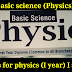Msbte I Scheme syllabus | First year for basic science ( Physics )

Semester I -Common to All Branches

(Electronics and telecommunication Engineering, Digital Electronics, industrial Electronics, instrumentation medical Electronics, Computer, Information technology )(MSBTE)

Here you will get the syllabus for physics for diploma students which are in (I scheme of Msbte)

Unit:-
1.Units and measurements
2.Electricity, Magnetism and semiconductor
3. Heat and optics

1. Units-I  Units and measurements

*Major learning outcomes

A. Describe the concept of given physical quantities with relevant unit of measurement.

B. Explain various systems of units and its need for the measurement of the given physical quantities Determine the dimensions of the given physical quantities

C. Determine the dimesdimen of the given physical quantities.

D. State the error in the given measurements with justification.

* Topics and subtopics

1. Units, physical quantities and their units

2. System of unit : CGS,MKS,FPS and SI

3. Dimesnion, dimensional formula

4. Errors, types of error : instrumental, systematic and random. Estimation of error Absolute, relative, and percentage error, significant error.

2. Unit II - Electricity Magnetism and semiconductor

*Major learning outcomes

A.Calculate electric field, potential and potential difference of the given static charge.
B. Describe the concept of given magnetic intensity and flux with relevant units.
C. Explain the heating effect of the given electric current.

D. Apply laws of series and parallel combination in the given electric circuits.

E.Distinguish the given conductors, semiconductors and insulators on the basis of energy bands.

F.Explain the I-V charactristicha and application of given p-n junction diode.

*Topics and subtopics

1.Concept of charge, Coulomb's inverse square law, Electric field, Electric field intensity. potential and potential difference

2. Magnet field and magnetic field intensity and its units, magnetic lines of force, magnetic flux.

3. Electric current, Ohm's law, specific resistance, laws of series and parallel combination of resistance, heating effecting of electric current.

4. A Conductors, Insulators and Semiconductors, Energy bands, intrinsic and extrinsic semiconductors.

5. P-N junction diode, l-V characteristics  p-n junction. applications of p-n junction diode.

3.Unit III (Heat and optics)

* Major learning outcomes

1. Convert the given I temperature in different temperature scales.

2.Describe the properties of the given good and bad conductors of heat.

3.Relate the characteristics of the three gas laws.

4.Determine the relation between specific heats tor the given materials.

5.Distinguish the phenomena of total internal reflection tor the given mediums.

6.Describe light propagation in the given type of optical fiber.

* Topics and subtopics

1. Heat, temperature, temperature scales.

2. Modes of transfer of heat. good and bad conductors of heat. law of thermal conductivity.

3. Boyle’s law, Charle's law, Gay Lussac's law, perfect gas equation.

4. Specific heat of gas at constant pressure and volume (CP and Cv),ratio of specific heats.

5. Reflection, refraction. laws of refraction. total internal reflection.

6. Optical fiber: Principle. construction and path of light through optical fiber. applications of optical fibers.

All the given point are related with the syllabus of physics I scheme

I scheme

Msbte I scheme

Msbte syllbus

New syllabus I scheme

Msbte I Scheme syllabus | First year for basic science ( Physics )Reviewed by Devid on September 26, 2018 Rating: 5